Definitions of Square Dance Calls and Concepts

Index -->  Plus  |  A1  |  A2  |  C1  |  C2  |  C3A  |  C3B  |  C4  |  NOL  |
Definitions (Text Only) -->  Plus  |  A1  |  A2  |  C1  |  C2  |  C3A  |  C3B  |  C4  |  NOL  |
 Find call:

Column \$B\$+\$i!%(B

1 \$BHVL\\$N?M\$O(B Trail Off \$B\$r\$7\$F(B Roll \$B\$r\$7(B, 2 \$BHVL\\$N?M\$O(B Circulate, Peel Off \$B\$r\$7\$F(B Roll \$B\$r\$7(B, 3 \$BHVL\\$H(B 4 \$BHVL\\$N?M\$O(B Circulate, Centers Cast Off 3/4 \$B\$r\$7(B, \$BA40w\$G(B Extend \$B\$r\$7\$^\$9!%(B

\$BJ?9T\$J(B Wave \$B\$G=*\$o\$j\$^\$9!%(B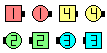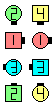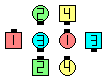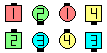Open Up The Column\$B\$NA0(B #1 Trail Off & Roll as #2 Circulate & Peel Off & Roll as #3 \$B\$H(B #4 Circulate(Open Up But Nothing)\$B\$N8e(B Centers Cast Off 3/4\$B\$N8e(B Extend\$B\$N8e(B (\$B=*\$o\$j(B)

\$BCmOpen Up The Column \$B\$O(B, \$B;O\$a\$N(B 2 \$B?M\$,(B Trail Off \$B\$N\$h\$&\$G\$J\$/(B, Trail & Peel \$B\$N\$h\$&\$KF0\$/\$3\$H\$r=|\$\$\$F\$O(B, Transfer The Column [A1] \$B\$HF1\$8\$G\$9!%(B \$B:#\$O;H\$o\$l\$F\$\$\$^\$;\$s\$,(B Clover the Column \$B\$H\$\$\$&%3!<%k\$,\$"\$j(B, \$B;O\$a\$N(B 2 \$B?M\$O(B Peel Off \$B\$NF0\$-(B (1/2 Invert The Column and 1/4 In) \$B\$r\$7\$^\$9!%(B \$B;d\$?\$A\$O(B, \$B\$3\$l\$i\$N(B 3 \$B\$D\$N%3!<%k\$r0lHL2=\$7(B, Trail (Transfer) The Column, Trail & Peel (Open Up) The Column, Peel (Clover) The Column and Peel & Trail The Column \$B\$,\$G\$-\$k\$H;W\$\$\$^\$9!%(B

Open Up And | But anything [C3A] (Lee Kopman 1976): Column \$B\$+\$i!%(B 1 \$BHVL\\$N?M\$O(B Trail Off \$B\$r\$7\$F(B Roll \$B\$r\$7(B, 2 \$BHVL\\$N?M\$O(B Circulate, Peel Off \$B\$r\$7\$F(B Roll \$B\$r\$7(B, 3 \$BHVL\\$H(B 4 \$BHVL\\$N?M\$O(B Circulate \$B\$r\$7\$F(B anything call \$B\$r\$7\$^\$9!%(B \$B\$3\$l\$O(B Transfer And anything [A2] \$B\$H;w\$F\$\$\$^\$9!%(B

\$B1~MQ(B:

• Magic Open Up The Column.
• 2 By 1 Open Up The Column: 3 \$B?M\$N(B Column \$B\$+\$i!%(B 1 \$BHVL\\$N?M\$O(B Trail Off \$B\$r\$7\$F(B Roll \$B\$r\$7(B, 2 \$BHVL\\$N?M\$O(B Circulate, Peel Off \$B\$r\$7\$F(B Roll \$B\$r\$7(B, 3 \$BHVL\\$N?M\$O(B Circulate \$B\$r\$7\$F(B Cast Off 3/4 \$B\$r\$7(B, Very Centers \$B\$,30B&\$N?M\$N4V\$X(B 'Extend' \$B\$r\$7\$^\$9!%(B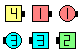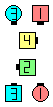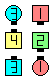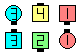2 By 1 Open Up The Column\$B\$NA0(B 2 By 1 Open Up But Nothing\$B\$N8e(B Centers Cast Off 3/4\$B\$N8e(B \$B30B&\$N?M\$N4V\$X(B 'Extend' \$B\$N8e(B (\$B=*\$o\$j(B)CALLERLAB definition for Open Up The ColumnChoreography for Open Up The ColumnComments? Questions? Suggestions?